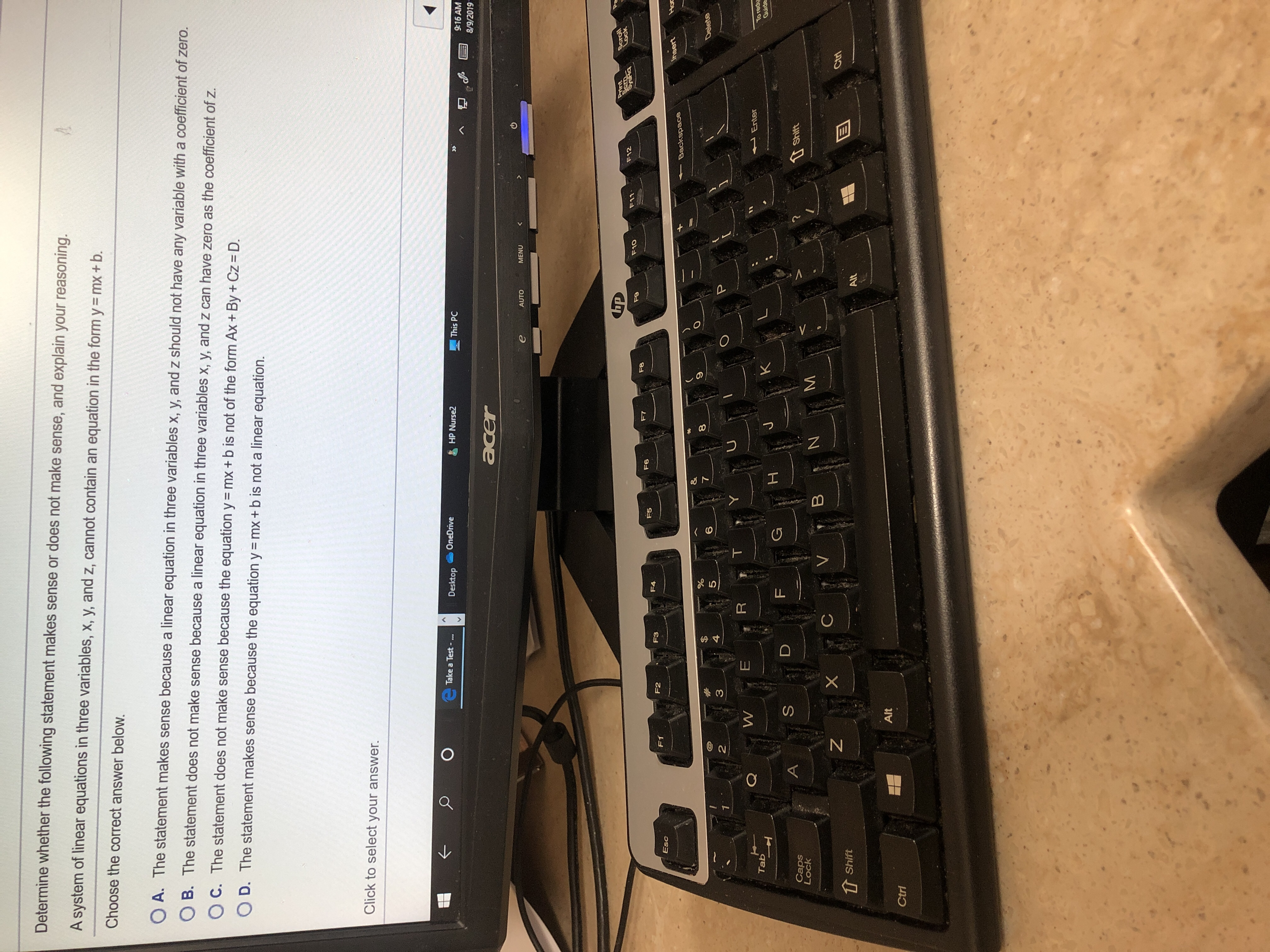Determine whether the following statement makes sense or does not make sense, and explain your reasoning.A system of linear equations in three variables, x, y, and z, cannot contain an equation in the form y= mx + b.Choose the correct answer below.O A. The statement makes sense because a linear equation in three variables x, y, and z should not have any variable with a coefficient of zero.O B. The statement does not make sense because a linear equation in three variables x, y, and z can have zero as the coefficient of z.O C. The statement does not make sense because the equation y mx + b is not of the form Ax + By + Cz = D.O D. The statement makes sense because the equation y = mx + b is not a linear equation.Click to select your answer.Take a Test -...OneDriveDesktopHP Nurse2This PC»9:16 AM8/9/2019acerMENUAUTOapScrollLockPrintSemSyeRaF12F11F10F9F8F7F6F5F4F3F2F1EscHoInsertBackspace%7Delete654321RWEnterTo reduGuideTabKJHGFDAShiftCapsLockBVCtriShiftAltAltCtriZLOUSN

Questionhelp_outlineImage TranscriptioncloseDetermine whether the following statement makes sense or does not make sense, and explain your reasoning. A system of linear equations in three variables, x, y, and z, cannot contain an equation in the form y= mx + b. Choose the correct answer below. O A. The statement makes sense because a linear equation in three variables x, y, and z should not have any variable with a coefficient of zero. O B. The statement does not make sense because a linear equation in three variables x, y, and z can have zero as the coefficient of z. O C. The statement does not make sense because the equation y mx + b is not of the form Ax + By + Cz = D. O D. The statement makes sense because the equation y = mx + b is not a linear equation. Click to select your answer. Take a Test -... OneDrive Desktop HP Nurse2 This PC » 9:16 AM 8/9/2019 acer MENU AUTO ap Scroll Lock Print Sem SyeRa F12 F11 F10 F9 F8 F7 F6 F5 F4 F3 F2 F1 Esc Ho Insert Backspace % 7 Delete 6 5 4 3 2 1 R W Enter To redu Guide Tab K J H G F D A Shift Caps Lock B V Ctri Shift Alt Alt Ctri Z LO US N fullscreen
Step 1

We can rewrite the equation y=mx...

Want to see the full answer?

See Solution

Want to see this answer and more?

Our solutions are written by experts, many with advanced degrees, and available 24/7

See Solution
Tagged in

Equations and In-equations